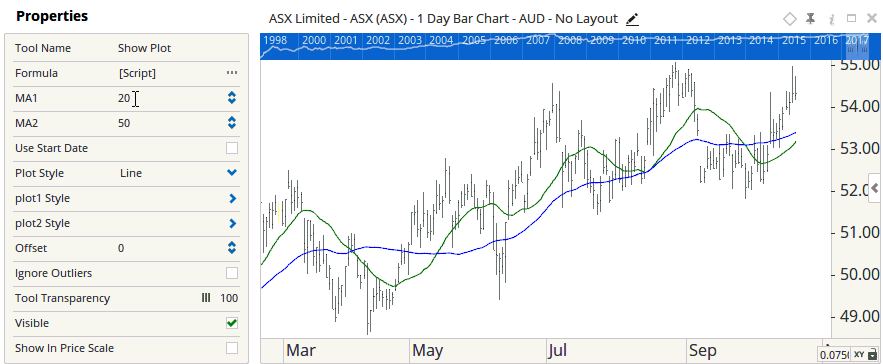# Bar Variables and Properties

Author: Optuma Team Last updated: Apr 3, 2020 05:19

## Overview

You can setup Bar = references to a variable, and display that variables value in the properties panel of the scripting tool, allowing it to be modified via the panel, rather than needing to edit the script each time.

### Bar Variables

To setup a bar variable script functions such as MA() can reference you use the \$ symbol.

For example:

``````\$a = 50;
``````

This sets the bar variable to 50. This can then be referenced in other areas of the script (anywhere a function contains a Bar= property.)

``````plot1 = MA(BARS = \$a);
``````

In this example the script would plot a 50SMA on the chart.

Bar Variables can be more than static numbers, they can be build from any script function, for example:

``````\$b = ATR(BARS = 100, MULT = 1);
\$c = Close() +100;
plot1 = MA(BARS = \$b);
plot2 = MA(BARS = \$c);
``````

In this example b would be the value of an ATR and c would be the value of the Closing price + 100.

### Bar Variables in Properties

When a bar variable has been setup in a script, you can add a property for this value to the tools properties panel. This is done using the “#” symbol.

For example:

``````#\$a = 50
``````

When looking at the properties of a tool containing this script, the value for a would be displayed and could be adjusted from the panel.This setup allows you to modify parts of the script like you would a standard tool, without the need to edit the underlying script code.Example script from above image:

``````#\$MA1 = 20;
#\$MA2 = 50;
plot1 = MA(Bars = \$MA1);
plot2 = MA(Bars = \$MA2);
``````